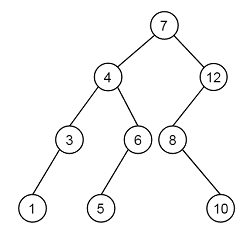Construct BST from its given level order traversal in C++

C++Server Side ProgrammingProgramming

Suppose we have one level order traversal. From this traversal. we have to generate the tree So if the traversal is like [7, 4, 12, 3, 6, 8, 1, 5, 10], then the tree will be like −To solve this, we will use recursive approach. The first element will be the root. The second element will be the left child, and third element will be right child (If the condition of BST satisfies), this property will be satisfied for all elements. So we will follow these steps −

• At first we have to take the first element of the array, and make this as root.

• Then take the second element. If that is smaller than root, then make it left child otherwise right child

• Then recursively call step 2 to make the BST.

Example

Live Demo

#include <iostream>
using namespace std;
class Node {
public:
int data;
Node *left, *right;
};
Node* getNode(int data) {
Node *newNode = new Node;
newNode->data = data;
newNode->left = newNode->right = NULL;
return newNode;
}
Node *lvlOrd(Node *root , int data) {
if(root==NULL){
root = getNode(data);
return root;
}
if(data <= root->data)
root->left = lvlOrd(root->left, data);
else
root->right = lvlOrd(root->right, data);
return root;
}
Node* makeTree(int arr[], int n) {
if(n==0)
return NULL;
Node *root= NULL;
for(int i=0;i<n;i++)
root = lvlOrd(root , arr[i]);
return root;
}
void inord(Node* root) {
if (!root)
return;
inord(root->left);
cout << root->data << " ";
inord(root->right);
}
int main() {
int arr[] = {7, 4, 12, 3, 6, 8, 1, 5, 10};
int n = sizeof(arr) / sizeof(arr);
Node *root = makeTree(arr, n);
cout << "Inorder Traversal: ";
inord(root);
}

Output

Inorder Traversal: 1 3 4 5 6 7 8 10 12